#### Maximum Likelihood Estimation Based on Laplace Approximation

##### Objective Function

Letdenote the vector of fixed-effects parameters andthe vector of covariance parameters. For Laplace estimation in the GLIMMIX procedure,includes the G-side parameters and a possible scale parameter, provided that the conditional distribution of the data contains such a scale parameter.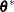is the vector of the G-side parameters.

The marginal distribution of the data in a mixed model can be expressed as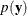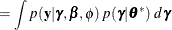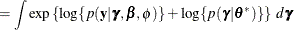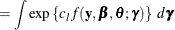If the constant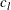is large, the Laplace approximation of this integral is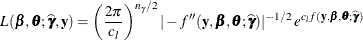where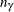is the number of elements in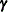,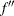is the second derivative matrix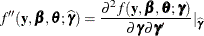and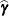satisfies the first-order condition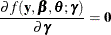The objective function for Laplace parameter estimation in the GLIMMIX procedure is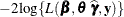. The optimization process is singly iterative, but becausedepends on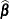and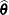, the GLIMMIX procedure solves a suboptimization problem to determine for given values ofandthe random-effects solution vector that maximizes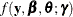.

When you have longitudinal or clustered data with m independent subjects or clusters, the vector of observations can be written as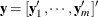, where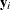is an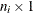vector of observations for subject (cluster) i (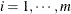). In this case, assuming conditional independence such that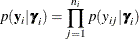the marginal distribution of the data can be expressed as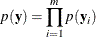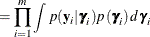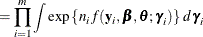where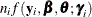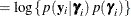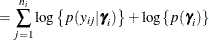When the number of observations within a cluster,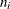, is large, the Laplace approximation to the ith individual’s marginal probability density function is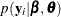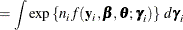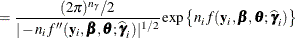where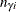is the common dimension of the random effects,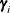. In this case, provided that the constant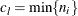is large, the Laplace approximation to the marginal log likelihood is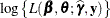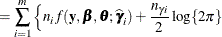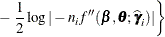which serves as the objective function for the METHOD=LAPLACE estimator in PROC GLIMMIX.

The Laplace approximation implemented in the GLIMMIX procedure differs from that in Wolfinger (1993) and Pinheiro and Bates (1995) in important respects. Wolfinger (1993) assumed a flat prior forand expanded the integrand aroundand, leaving only the covariance parameters for the overall optimization. The fixed effectsand the random effectsare determined in a suboptimization that takes the form of a linear mixed model step with pseudo-data. The GLIMMIX procedure involves only the random effects vectorin the suboptimization. Pinheiro and Bates (1995) and Wolfinger (1993) consider a modified Laplace approximation that replaces the second derivative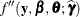with an (approximate) expected value, akin to scoring. The GLIMMIX procedure does not use an approximation to. The METHOD=RSPL estimates in PROC GLIMMIX are equivalent to the estimates obtained with the modified Laplace approximation in Wolfinger (1993). The objective functions of METHOD=RSPL and Wolfinger (1993) differ in a constant that depends on the number of parameters.

##### Asymptotic Properties and the Importance of Subjects

Suppose that the GLIMMIX procedure processes your data by subjects (see the section Processing by Subjects) and letdenote the number of observations per subject,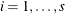. Arguments in Vonesh (1996) show that the maximum likelihood estimator based on the Laplace approximation is a consistent estimator to order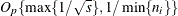. In other words, as the number of subjects and the number of observations per subject grows, the small-sample bias of the Laplace estimator disappears. Note that the term involving the number of subjects in this maximum relates to standard asymptotic theory, and the term involving the number of observations per subject relates to the accuracy of the Laplace approximation (Vonesh, 1996). In the case where random effects enter the model linearly, the Laplace approximation is exact and the requirement that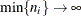can be dropped.

If your model is not processed by subjects but is equivalent to a subject model, the asymptotics with respect to s still apply, because the Hessian matrix of the suboptimization forbreaks into s separate blocks. For example, the following two models are equivalent with respect to s and, although only for the first model does PROC GLIMMIX process the data explicitly by subjects:

proc glimmix method=laplace;
class sub A;
model y = A;
random intercept / subject=sub;
run;

proc glimmix method=laplace;
class sub A;
model y = A;
random sub;
run;


The same holds, for example, for models with independent nested random effects. The following two models are equivalent, and you can derive asymptotic properties related to s and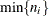from the model in the first run:

proc glimmix method=laplace;
class A B block;
model y = A B A*B;
random intercept A / subject=block;
run;

proc glimmix method=laplace;
class A B block;
model y = A B A*B;
random block a*block;
run;


The Laplace approximation requires that the dimension of the integral does not increase with the size of the sample. Otherwise the error of the likelihood approximation does not diminish with. This is the case, for example, with exchangeable arrays (Shun and McCullagh, 1995), crossed random effects (Shun, 1997), and correlated random effects of arbitrary dimension (Raudenbush, Yang, and Yosef, 2000). Results in Shun (1997), for example, show that even in this case the standard Laplace approximation has smaller bias than pseudo-likelihood estimates.